# Test: Single Correct MCQs: Properties of Triangle | JEE Advanced

## 19 Questions MCQ Test Maths 35 Years JEE Main & Advanced Past year Papers | Test: Single Correct MCQs: Properties of Triangle | JEE Advanced

Description
Attempt Test: Single Correct MCQs: Properties of Triangle | JEE Advanced | 19 questions in 40 minutes | Mock test for JEE preparation | Free important questions MCQ to study Maths 35 Years JEE Main & Advanced Past year Papers for JEE Exam | Download free PDF with solutions
QUESTION: 1

### If the bisector of the angle P of a triangle PQR meets QR in S, then

Solution:

QS : SR = PQ : PR (as bisector of an angle, in a triangle, divides the opposite side in the same ratio as the sides containing the angle.)

QUESTION: 2

### From the top of a light-house 60 metres high with its base at the sea-level, the angle of depression of a boat is 15°. The distance of the boat from the foot of the light house is

Solution: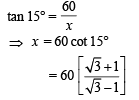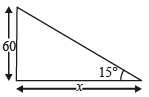QUESTION: 3

### In a triangle ABC, angle A is greater than angle B. If the measures of angles A and B satisfy the equation 3 sin x – 4 sin3x – k = 0, 0 < k < 1, then the measure of angle C is

Solution:

Given that A > B and 3 sin x – 4 sin 3 x – k = 0, 0 <  k < 1
⇒ sin 3 x = k
As A and B satisfy above eq. (given)
∴ sin 3A = k,  sin 3B = k
⇒ sin 3A – sin 3B = 0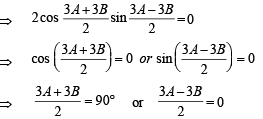⇒ A + B = 60°  or  A = B
But given that A  >  B,   ∴  A ≠ B
Thus, A + B = 60°
But A + B + C = 180°  ⇒ C = 180° –  60° =  120°
∴ C = 2π3

QUESTION: 4

If the lengths of the sides of triangle are 3, 5, 7 then the largest angle of the triangle is

Solution:

Let  a = 3, b = 5, c = 7 then the largest ∠ is  opp. to the longest side, i.e., ∠C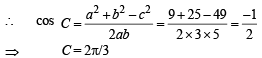QUESTION: 5

In a triangle ABC,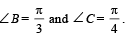Let D divide BC internally in the ratio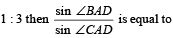Solution: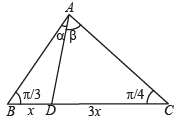In ΔABD, applying Sine law we get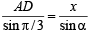⇒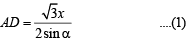In ΔACD, applying Sine law, we get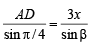⇒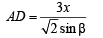....(2)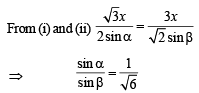QUESTION: 6

In a triangle ABC,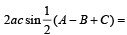Solution:

We know that A + B + C = 180°
⇒ A + C – B = 180° – 2B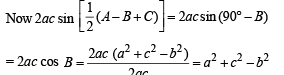QUESTION: 7

In a triangle ABC, let ∠C = π/2. If r is the inradius and R is the circumradius of the triangle, then 2(r + R) is equal to

Solution:

We know by Sine rule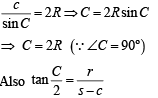⇒ r = s - c (∴ ∠C = 90º)
⇒ a + b - c= 2r
or   2r + c = a+b
or   2r +2R = a+b (Using C = 2R)

QUESTION: 8

A pole stands vertically inside a triangular park ΔABC. If the angle of elevation of the top of the pole from each corner of the park is same, then in ΔABC the foot of the pole is at the

Solution: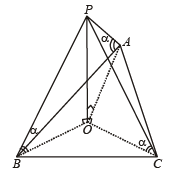Let OP = Pole,
∠PAO = ∠PBO = ∠PCQ = α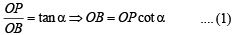Similarly OA = OP  cot a .... (2)
Similarly OC = OP  cot a .... (3)
From  (1),  (2)  and (3), OA = OB = OC ⇒ O  is the point of circumcentre of the triangle ABC.

QUESTION: 9

A man from the top of a 100 metres high tower sees a car moving towards the tower at an angle of depression of 30°. After some time, the angle of depression becomes 60°. The distance (in metres) travelled by the car during this time is

Solution: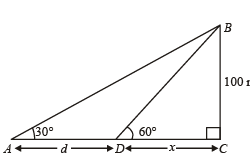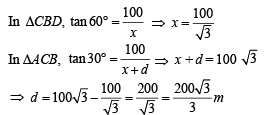QUESTION: 10

Which of the following pieces of data does NOT uniquely determine an acute-angled triangle ABC (R being the radius of the circumcircle)?

Solution:

We know by Sine law in ΔABC as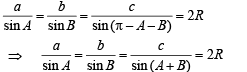(a) If we know a  , sin A  , sin B we can find b , c ; values of ∠’s  A , B and C all.
(b) Using a , b , c we can find ∠A  , ÐB, ∠C using cosine law.
(c) a , sin B , R are given then sin A , b and hence sin (A + B) and then C can be found.
(d) If we know a , sin A , R then we know  only the ratio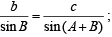we can  not determine the values of b , c , sin B , sin C seperately.
∴  D can not be determined in  this case.

QUESTION: 11

If the angles of a triangle are in the ratio 4 : 1 : 1, then the ratio of the longest side to the perimeter is

Solution:

Given that  A : B : C = 4 : 1 : 1
Let A = 4x,  B =  x , C = x
But A + B + C = 180°
∴ 4x + x + x = 180°  ⇒ x = 30°
∴ A = 120° ,  B = 30° , C  = 30°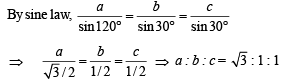∴ Ratio of longest side to the perimeter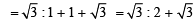QUESTION: 12

The sides of a triangle are in th e rat io 1 : √3 : 2, then the angles of the triangle are in the ratio

Solution:

Sides are in the Ratio 1 : √3 :2

Let a = k , b = √3 k and c = 2k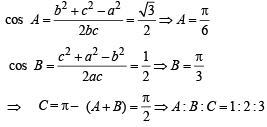QUESTION: 13

In an equil ateral triangle, 3 coins of radii 1 unit each are kept so that they touch each other and also the sides of the triangle. Area of the triangle is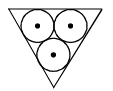Solution:

The situation is as shown in the figure. For circle with centre C2, BP and BP' are two tangents from B to circle, therefore BC2 must be the ∠bisector of ∠B. But ∠B = 60° (∴ ΔABC is an equilateral Δ)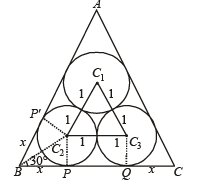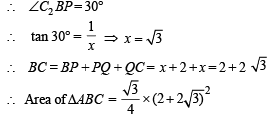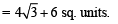QUESTION: 14

In a triangle ABC, a, b, c are the lengths of its sides and A, B, C are the angles of triangle ABC. The correct  relation is given by

Solution:

Let us consider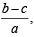which is involved in each of the these options.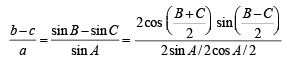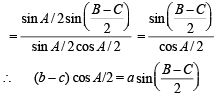QUESTION: 15

One angle of an isosceles Δ is 120º and radius of its incircle = √3 . Then the area of the triangle in sq. units is

Solution:

By Sine law in ΔABC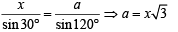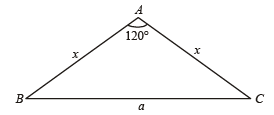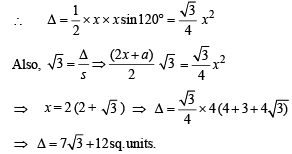QUESTION: 16

Let ABCD be a quadrilateral with area 18, with side AB parallel to the side CD and 2AB = CD. Let AD be perpendicular to AB and CD. If a circle is drawn inside the quadrilateral ABCD touching all the sides, then its radius is

Solution:

Given AB || CD,  CD = 2AB Let AB = a then CD = 2a Let radius of circle be r.
Let circle touches AB at P, BC at Q, AD at R and CD at S.
Then AR = AP = r, BP = BQ = a – r
DR = DS = r  and CQ = CS = 2a – r
In ΔBEC BC2 = BE2 + EC2 ⇒ (a – r + 2a – r)2 = (2r)2 + (a)2
⇒  9a2 + 4r2 – 12ar = 4r2 + a2

⇒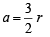....(1)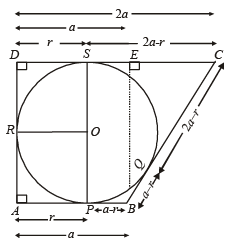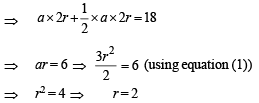QUESTION: 17

If the angles A, B and C of a triangle are in an arithmetic progression and if a, b and c denote the lengths of the sides opposite to A, B and C respectively, then the value of the expression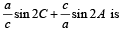Solution:

∵ A, B, C are in A.P..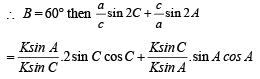= 2(sin A cos C + cos A sin C ) =2 sin( A + C )=2 sin B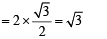QUESTION: 18

Let PQR be a triangle of area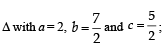where a, b, and c are the lengths of the sides of the triangle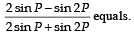Solution:

We have,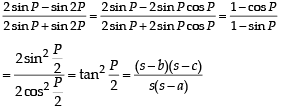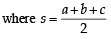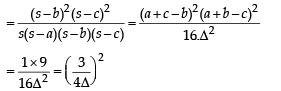QUESTION: 19

In a triangle the sum of two sides is x and the product of the same sides is y. If x2 – c2 = y, where c is the third side of the triangle, then the ratio of the in radius to the circum-radius of the triangle is

Solution:

Let two sides of Δ be a and b.
Then a + b = x and ab = y
Also given x2 – c2 = y, where c is the third side of Δ.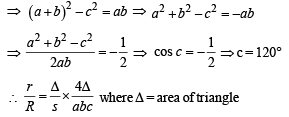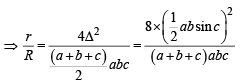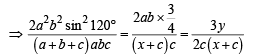Use Code STAYHOME200 and get INR 200 additional OFF Use Coupon Code